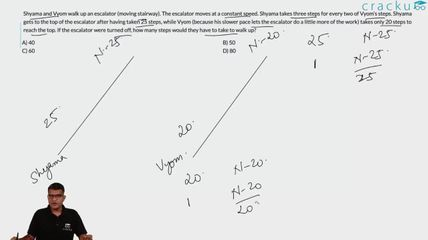Question 29

# Shyama and Vyom walk up an escalator (moving stairway). The escalator moves at a constant speed. Shyama takes three steps for every two of Vyom’s steps. Shyama gets to the top of the escalator after having taken 25 steps, while Vyom (because his slower pace lets the escalator do a little more of the work) takes only 20 steps to reach the top. If the escalator were turned off, how many steps would they have to take to walk up?

Solution

Let the number of steps on the escalator be x.

So, by the time Shyama covered 25 steps, the escalator moved 'x-25' steps.

Hence, the ratio of speeds of Shyama and escalator = 25:(x-25)

Similarly, the ratio of speeds of Vyom and escalator = 20:(x-20)

But the ratio is 3:2

Ratio of speeds of Shyama and Vyom = 25(x-20)/20*(x-25) = 3/2

=> 10(x-20) = 12(x-25)

=> 2x = 100 => x = 50

### View Video Solution